# Learning Electronics

Learn to build electronic circuits

# Zener diodes

### Question 1:

 Don't just sit there! Build something!!

Learning to mathematically analyze circuits requires much study and practice. Typically, students practice by working through lots of sample problems and checking their answers against those provided by the textbook or the instructor. While this is good, there is a much better way.
You will learn much more by actually building and analyzing real circuits, letting your test equipment provide the änswers" instead of a book or another person. For successful circuit-building exercises, follow these steps:

1.
Carefully measure and record all component values prior to circuit construction, choosing resistor values high enough to make damage to any active components unlikely.
2.
Draw the schematic diagram for the circuit to be analyzed.
3.
Carefully build this circuit on a breadboard or other convenient medium.
4.
Check the accuracy of the circuit's construction, following each wire to each connection point, and verifying these elements one-by-one on the diagram.
5.
Mathematically analyze the circuit, solving for all voltage and current values.
6.
Carefully measure all voltages and currents, to verify the accuracy of your analysis.
7.
If there are any substantial errors (greater than a few percent), carefully check your circuit's construction against the diagram, then carefully re-calculate the values and re-measure.

When students are first learning about semiconductor devices, and are most likely to damage them by making improper connections in their circuits, I recommend they experiment with large, high-wattage components (1N4001 rectifying diodes, TO-220 or TO-3 case power transistors, etc.), and using dry-cell battery power sources rather than a benchtop power supply. This decreases the likelihood of component damage.
As usual, avoid very high and very low resistor values, to avoid measurement errors caused by meter "loading" (on the high end) and to avoid transistor burnout (on the low end). I recommend resistors between 1 kW and 100 kW.
One way you can save time and reduce the possibility of error is to begin with a very simple circuit and incrementally add components to increase its complexity after each analysis, rather than building a whole new circuit for each practice problem. Another time-saving technique is to re-use the same components in a variety of different circuit configurations. This way, you won't have to measure any component's value more than once.
Let the electrons themselves give you the answers to your own "practice problems"!

Notes:
It has been my experience that students require much practice with circuit analysis to become proficient. To this end, instructors usually provide their students with lots of practice problems to work through, and provide answers for students to check their work against. While this approach makes students proficient in circuit theory, it fails to fully educate them.
Students don't just need mathematical practice. They also need real, hands-on practice building circuits and using test equipment. So, I suggest the following alternative approach: students should build their own "practice problems" with real components, and try to mathematically predict the various voltage and current values. This way, the mathematical theory "comes alive," and students gain practical proficiency they wouldn't gain merely by solving equations.
Another reason for following this method of practice is to teach students scientific method: the process of testing a hypothesis (in this case, mathematical predictions) by performing a real experiment. Students will also develop real troubleshooting skills as they occasionally make circuit construction errors.
Spend a few moments of time with your class to review some of the "rules" for building circuits before they begin. Discuss these issues with your students in the same Socratic manner you would normally discuss the worksheet questions, rather than simply telling them what they should and should not do. I never cease to be amazed at how poorly students grasp instructions when presented in a typical lecture (instructor monologue) format!
A note to those instructors who may complain about the "wasted" time required to have students build real circuits instead of just mathematically analyzing theoretical circuits:
What is the purpose of students taking your course?
If your students will be working with real circuits, then they should learn on real circuits whenever possible. If your goal is to educate theoretical physicists, then stick with abstract analysis, by all means! But most of us plan for our students to do something in the real world with the education we give them. The "wasted" time spent building real circuits will pay huge dividends when it comes time for them to apply their knowledge to practical problems.
Furthermore, having students build their own practice problems teaches them how to perform primary research, thus empowering them to continue their electrical/electronics education autonomously.
In most sciences, realistic experiments are much more difficult and expensive to set up than electrical circuits. Nuclear physics, biology, geology, and chemistry professors would just love to be able to have their students apply advanced mathematics to real experiments posing no safety hazard and costing less than a textbook. They can't, but you can. Exploit the convenience inherent to your science, and get those students of yours practicing their math on lots of real circuits!

### Question 2:

Shown here is the characteristic curve of a diode: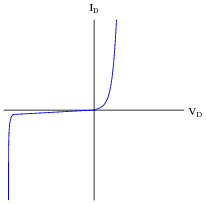Identify which area of this curve represents normal forward-biased operation, and which represents reverse-biased operation. Also, explain the significance of the near-vertical portion of the curve in the lower-left quadrant of the graph.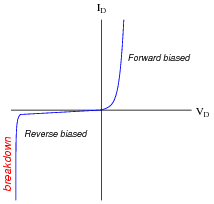Challenge question: identify where the diode's reverse saturation current may be found on this graph.

Notes:
Ask your students to identify the region of the curve described by Shockley's diode equation. The exponential form of that equation really only models one definite portion of the curve!

### Question 3:

How does the behavior of a zener diode differ substantially from that of a normal (rectifying) diode?
Zener diodes break down at substantially lower reverse voltages than rectifying diodes, and their breakdown voltages are predictable.

Notes:
Ask students what zener diodes would likely be used for. Why would we need or desire a device with a stable breakdown voltage?

### Question 4:

Not all "zener" diodes break down in the exact same manner. Some operate on the principle of zener breakdown, while others operate on the principle of avalanche breakdown. How do the temperature coefficients of these two zener diode types compare, and how are you able to discern whether a zener diode uses one principle or the other just from its breakdown voltage rating?
Correspondingly, is there a way we could determine the type of breakdown action from experimental measurements on a zener diode? Explain how such an experiment might be set up.
Low-voltage zener diodes have negative temperature coefficients, because they exploit the zener effect. High-voltage zener diodes have positive temperature coefficients, because they exploit the avalanche effect. I'll let you research how to tell the difference between zener diodes employing each phenomenon on your own!
Challenge question: explain the difference between the "zener" and ävalanche" effects, in terms of charge carrier action.

Notes:
Regular "rectifying" diodes also have temperature coefficients. Ask your students to identify whether the temperature coefficient for a rectifying diode is typically positive or negative, and what this actually means. It is very easy to experimentally verify this, so you may want to ask your students to demonstrate how to determine the sign of a rectifying diode's temperature coefficient as a prelude to reviewing the experimental portion of the original question.
Ask your students to identify the typical voltage values associated with both types of breakdown effect. This will quickly reveal which students did their research for this question, as opposed to those who merely read the answer given here!

### Question 5:

Explain how the characteristic curve of a 24 volt zener diode (as plotted by a curve tracer) differs from that of a normal rectifying diode, shown here: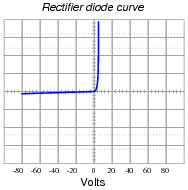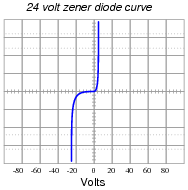Notes:
The purpose of this question is to cause students to think about what a characteristic curve means, in the context of diode comparisons. The breakdown voltage of a zener diode is typically so low compared to that of a normal rectifying diode that this region may be easily shown on the curve tracer screen.

### Question 6:

Suppose you had the boring job of manually maintaining the output voltage of a DC generator constant. Your one and only control over voltage is the setting of a rheostat: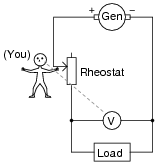What would you have to do to maintain the load voltage constant if the load resistance changed so as to draw more current? Being that your only control over load voltage is the adjustment of a variable resistance in series with the generator, what does this imply about the generator's output voltage (directly across the generator terminals), compared to the target load voltage?
In order to increase the load voltage, you must decrease the resistance of the rheostat. In order for this scheme to work, the generator's voltage must be greater than the target load voltage.
Note: this general voltage control scheme is known as series regulation, where a series resistance is varied to control voltage to a load.

Notes:
The direction of rheostat adjustment should be obvious, as is the fact that the generator's voltage must be at least as high as the intended (target) load voltage. However, it may not be obvious to all that the generator's voltage cannot merely be equal to the intended load voltage.
To illustrate the necessity of this, ask your students how the system would work if the generator's output voltage was exactly equal to the intended load voltage. Emphasize the fact that the generator is not perfect: it has its own internal resistance, the value of which cannot be changed by you. What position would the rheostat have to be in, under these conditions, in order to maintain target voltage at the load? Could the target voltage be maintained at all?

### Question 7:

Suppose you had the boring job of manually maintaining the output voltage of a DC generator constant. Your one and only control over voltage is the setting of a rheostat: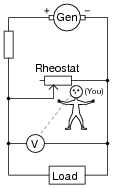What would you have to do to maintain the load voltage constant if the load resistance changed so as to draw more current? Being that your only control over load voltage is the adjustment of a variable resistance in parallel with the load, what does this imply about the generator's output voltage (directly across the generator terminals), compared to the target load voltage?
In order to increase the load voltage, you must increase the resistance of the rheostat. In order for this scheme to work, the generator's voltage must be greater than the target load voltage.
Note: this general voltage control scheme is known as shunt regulation, where a parallel (shunt) resistance is varied to control voltage to a load.
Follow-up question: assuming the load voltage is maintained at a constant value by an astute rheostat operator despite fluctuations in load current, how would you characterize the current through the generator's windings? Does it increase with load current, decrease with load current, or remain the same? Why?

Notes:
The direction of rheostat adjustment should be obvious, as is the fact that the generator's voltage must be at least as high as the intended (target) load voltage. However, it may not be obvious to all that the generator's voltage cannot merely be equal to the intended load voltage.
To illustrate the necessity of this, ask your students how the system would work if the generator's output voltage was exactly equal to the intended load voltage. Emphasize the fact that the generator is not perfect: it has its own internal resistance, the value of which cannot be changed by you. What position would the rheostat have to be in, under these conditions, in order to maintain target voltage at the load? Could the target voltage be maintained at all?
A helpful analogy for students is that of a car with an automatic transmission, with its speed being controlled by the brake pedal while the accelerator pedal is maintained at a constant position. This is not the most energy-efficient method of speed control, but it will work within certain limits!

### Question 8:

Describe how a zener diode is able to maintain regulated (nearly constant) voltage across the load, despite changes in load current: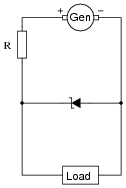The zener draws more or less current as necessary from the generator (through the series resistor) to maintain voltage at a nearly constant value.
Follow-up question #1: if the generator happens to output some ripple voltage (as all electromechanical DC generators do), will any of that ripple voltage appear at the load, after passing through the zener diode voltage regulator circuit?
Follow-up question #2: would you classify the zener diode in this circuit as a series voltage regulator or a shunt voltage regulator? Explain your answer.
Challenge question: at what point is the zener diode unable to regulate load voltage? Is there some critical load condition at which the diode ceases to regulate voltage?

Notes:
Ask your students to describe how energy-efficient they think this circuit is. Do they suspect it would be more suitable for low-current applications or high-current applications?

### Question 9:

Calculate the current through the zener diode for the given values of load resistance in this circuit: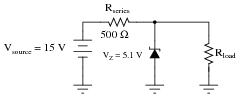Rload = 1 kW ; Izener =
Rload = 910 W ; Izener =
Rload = 680 W ; Izener =
Rload = 470 W ; Izener =
Rload = 330 W ; Izener =

Do you see any relationship between load current and zener diode current? If so, explain what that relationship is.
As the load current increases (with less load resistance), zener diode current decreases:

Rload = 1 kW ; Izener = 14.7 mA
Rload = 910 W ; Izener = 14.2 mA
Rload = 680 W ; Izener = 12.3 mA
Rload = 470 W ; Izener = 8.95 mA
Rload = 330 W ; Izener = 4.35 mA

Follow-up question: what value of load resistance will result in zero current through the zener diode (while still maintaining an output voltage of 5.1 volts)?

Notes:
This exercise in current calculation is supposed to get students to realize the inverse relationship between load current and zener current: that the zener diode regulates voltage by acting as a parasitic load of varying proportion. Simply put, the diode loads down the circuit as much as needed to maintain a stable voltage at the load terminals.
It should be noted that the calculated answers shown here will not precisely match a real zener diode circuit, due to the fact that zener diodes tend to gradually taper off in current as the applied voltage nears the zener voltage rating rather than current sharply dropping to zero as a simpler model would predict.
The follow-up question is very important. All zener diode regulator circuits have a minimum load resistance value that must be adhered to, lest the output voltage droop below the regulation point. Discuss with your students how the zener diode's "loading" behavior explains the need for a certain minimum load resistance value.

### Question 10:

Calculate the current through the zener diode for the given values of load resistance in this circuit:Rload = 1.5 kW ; Izener =
Rload = 1 kW ; Izener =
Rload = 910 W ; Izener =
Rload = 780 W ; Izener =

Rload = 1.5 kW ; Izener = 8.15 mA
Rload = 1 kW ; Izener = 5.52 mA
Rload = 910 W ; Izener = 4.74 mA
Rload = 780 W ; Izener = 3.29 mA

Follow-up question: what value of load resistance will result in zero current through the zener diode (while still maintaining an output voltage of 7.9 volts)?

Notes:
This exercise in current calculation is supposed to get students to realize the inverse relationship between load current and zener current: that the zener diode regulates voltage by acting as a parasitic load of varying proportion. Simply put, the diode loads down the circuit as much as needed to maintain a stable voltage at the load terminals.
It should be noted that the calculated answers shown here will not precisely match a real zener diode circuit, due to the fact that zener diodes tend to gradually taper off in current as the applied voltage nears the zener voltage rating rather than current sharply dropping to zero as a simpler model would predict.
The follow-up question is very important. All zener diode regulator circuits have a minimum load resistance value that must be adhered to, lest the output voltage droop below the regulation point. Discuss with your students how the zener diode's "loading" behavior explains the need for a certain minimum load resistance value.

### Question 11:

Calculate the current through the zener diode for the given values of load resistance in this circuit: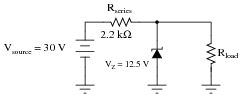Rload = 2 kW ; Izener =
Rload = 3 kW ; Izener =
Rload = 4 kW ; Izener =
Rload = 5 kW ; Izener =

Rload = 2 kW ; Izener = 1.705 mA
Rload = 3 kW ; Izener = 3.788 mA
Rload = 4 kW ; Izener = 4.830 mA
Rload = 5 kW ; Izener = 5.455 mA

Follow-up question: what value of load resistance will result in zero current through the zener diode (while still maintaining an output voltage of 5.1 volts)?

Notes:
This exercise in current calculation is supposed to get students to realize the inverse relationship between load current and zener current: that the zener diode regulates voltage by acting as a parasitic load of varying proportion. Simply put, the diode loads down the circuit as much as needed to maintain a stable voltage at the load terminals.
It should be noted that the calculated answers shown here will not precisely match a real zener diode circuit, due to the fact that zener diodes tend to gradually taper off in current as the applied voltage nears the zener voltage rating rather than current sharply dropping to zero as a simpler model would predict.
The follow-up question is very important. All zener diode regulator circuits have a minimum load resistance value that must be adhered to, lest the output voltage droop below the regulation point. Discuss with your students how the zener diode's "loading" behavior explains the need for a certain minimum load resistance value.

### Question 12:

Calculate the power dissipated by the 5-volt zener diode for the following values of motor current (assume the battery voltage remains constant at 12 volts):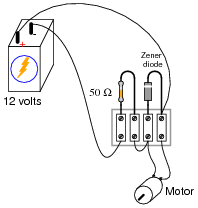Imotor = 20 mA ; Pzener =
Imotor = 50 mA ; Pzener =
Imotor = 90 mA ; Pzener =
Imotor = 120 mA ; Pzener =
Imotor = 150 mA ; Pzener =

Imotor = 20 mA ; Pzener = 600 mW
Imotor = 50 mA ; Pzener = 450 mW
Imotor = 90 mA ; Pzener = 250 mW
Imotor = 120 mA ; Pzener = 100 mW
Imotor = 150 mA ; Pzener = 0 mW

Follow-up question: is load voltage maintained at 5 volts constant throughout this range of load currents (from 20 mA to 150 mA)?

Notes:
The follow-up question is fairly important here, as students need to realize the limitations of zener-based voltage regulators. Most importantly, are they able to calculate the exact current limit of a zener-based voltage regulator - the point at which it stops regulating?
It should be noted that the calculated answers shown here will not precisely match a real zener diode circuit, due to the fact that zener diodes tend to gradually taper off in current as the applied voltage nears the zener voltage rating rather than current sharply dropping to zero as a simpler model would predict.

### Question 13:

Calculate the current through the zener diode for the given values of input (source) voltage in this circuit:Vsource = 25 V ; Izener =
Vsource = 20 V ; Izener =
Vsource = 15 V ; Izener =
Vsource = 10 V ; Izener =
Vsource = 5 V ; Izener =

Do you see any relationship between source voltage and zener diode current? If so, explain what that relationship is.
As the source voltage decreases, zener diode current also decreases:

Vsource = 25 V ; Izener = 41.49 mA
Vsource = 20 V ; Izener = 30.85 mA
Vsource = 15 V ; Izener = 20.21 mA
Vsource = 10 V ; Izener = 9.58 mA
Vsource = 5 V ; Izener = 0 mA

Follow-up question: what value of source voltage input will result in zero current through the zener diode (while still maintaining an output voltage of 5.1 volts)?

Notes:
This exercise in current calculation is supposed to get students to realize the inverse relationship between input voltage and zener current: that the zener diode regulates voltage by acting as a parasitic load of varying proportion. Simply put, the diode loads down the circuit as much as needed to maintain a stable voltage at the load terminals.
It should be noted that the calculated answers shown here will not precisely match a real zener diode circuit, due to the fact that zener diodes tend to gradually taper off in current as the applied voltage nears the zener voltage rating rather than current sharply dropping to zero as a simpler model would predict.
The follow-up question is very important. All zener diode regulator circuits have a minimum input voltage value that must be adhered to, lest the output voltage droop below the regulation point. Discuss with your students how the zener diode's "loading" behavior explains the need for a certain minimum source voltage.

### Question 14:

Qualitatively determine what will happen to the series resistor current and the zener diode current in this voltage regulator circuit if the load current suddenly decreases. Assume that the zener diode's behavior is ideal; i.e. its voltage drop holds absolutely constant throughout its operating range.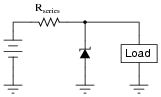IRseries = (increase, decrease, or unchanged?)
Izener = (increase, decrease, or unchanged?)
If the load current decreases, Izener will increase and IRseries will remain unchanged.
Challenge question: what do you think will happen with a real zener diode, where its voltage drop does change slightly with changes in current?

Notes:
A conceptual understanding of zener diode regulator circuits is important, perhaps even more important than a quantitative understanding. Your students will need to understand what happens to the different variables in such a circuit when another parameter changes, in order to understand how these circuits will dynamically react to changing load or source conditions.

### Question 15:

Qualitatively determine what will happen to the load current and the zener diode current in this voltage regulator circuit if the source voltage suddenly increases. Assume that the zener diode's behavior is ideal; i.e. its voltage drop holds absolutely constant throughout its operating range.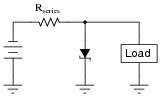Iload = (increase, decrease, or unchanged?)
Izener = (increase, decrease, or unchanged?)
If the source voltage increases, Izener will increase and Iload will remain unchanged.
Challenge question: what do you think will happen with a real zener diode, where its voltage drop does change slightly with changes in current?

Notes:
A conceptual understanding of zener diode regulator circuits is important, perhaps even more important than a quantitative understanding. Your students will need to understand what happens to the different variables in such a circuit when another parameter changes, in order to understand how these circuits will dynamically react to changing load or source conditions.

### Question 16:

Zener diodes are simple and useful devices for building voltage regulator circuits, but are times when you may have to improvise in the absence of the proper zener diode. Explain how normal diodes might be used as crude substitutes for a zener diode in the following circuit: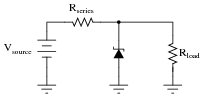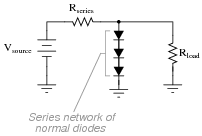Notes:
I have actually done this before in home-made circuitry. Voltage regulation isn't that good (especially the temperature dependence), but it is better than no regulation at all!

### Question 17:

Suppose you needed to build a simple voltage regulator circuit with a regulation point of 4.5 volts, but had no zener diodes to work with. Can you think of a way normal diodes could be used for the purpose instead?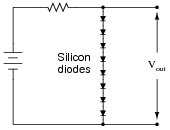(The number of series-connected diodes required depends on the actual forward voltage drop of each diode under loaded conditions.)

Notes:
Some students may suggest to use normal diodes backwards, exploiting the reverse-breakdown phenomenon common to all PN junctions. Whether or not this suggestion is made, ask your students why it would not be a practical solution in this case.

### Question 18:

At what load resistance value will this voltage regulator circuit begin to lose its ability to regulate voltage? Also, determine whether the voltage regulation is lost for load resistance values greater than this threshold value, or less than this threshold value.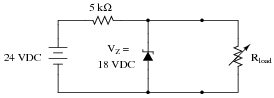There will be no load voltage regulation for any load resistance values less than 15 kW.
Follow-up question: calculate the power dissipated by all components in this circuit, if Rload = 30 kW.
Challenge question: write an equation solving for the minimum load resistance required to maintain voltage regulation.

Notes:
For those students struggling with the "greater than"/"less than" issue, suggest to them that they imagine the load resistance assuming extreme values: first 0 ohms, and then infinite ohms. After they do this, ask them to determine under which of these extreme conditions is the load voltage regulation still maintained.
Performing "thought experiments" with extreme component values is a highly effective problem-solving technique for many applications, and is one you should stress to your students often.
It should be noted that the calculated answer shown here will not precisely match a real zener diode circuit, due to the fact that zener diodes tend to gradually taper off in current as the applied voltage nears the zener voltage rating rather than current sharply dropping to zero as a simpler model would predict.

### Question 19:

Prior to the advent of zener diodes, gas-discharge tubes and bulbs were commonly used as voltage regulating devices.Explain how such a device regulates voltage, and comment on whether or not this type of device is still practical in modern circuit design.
Gas discharge devices, like zener diodes, exploit the sharply vertical portions of their current/voltage transfer functions to regulate voltage over a wide range of current: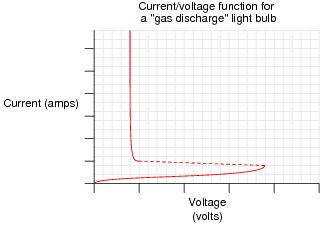Notes:
The gas discharge lamp's transfer function may be confusing to analyze at first, but it makes sense once students recall the principle of gas ionization with increasing voltage. Ask them to explain what the significance of each graph's vertical portions is, in the context of voltage regulation.

### Question 20:

Precision voltage reference regulators are often constructed of two zener diodes connected in series like this: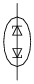Explain why two zener diodes provide greater stability than a single zener diode would, and also draw a circuit showing a voltage source, so this component functions as a complete voltage reference.
Two zener diodes provide better stability than a single zener diode because the thermal coefficients of the diodes in their respective modes are complementary. This assumes, of course, that the reverse-biased diode uses the avalanche effect to regulate voltage.
A functioning voltage regulator circuit might look like this:Notes:
Some students may become confused by the word "complementary" as it is used in the answer. Ask all your students to explain what this word means, in the context of two temperature coefficients and increased stability.

### Question 21:

It is possible to purchase oven-stabilized zener ICs (integrated circuits). Explain what these are, and what they are useful for.
Oven-stabilized zeners are used for precision voltage references. I'll let you research how they are constructed and how they function.

Notes:
Challenge your students to show you a datasheet for one of these devices!

### Question 22:

 �f(x)  dx   Calculus alert!

How is the parameter of zener impedance defined for a zener diode? Should an ideal zener diode have a zener impedance figure equal to zero, or infinite? Why?
Zzener = [(DEdiode)/(DIdiode)]    or    Zzener = [(d Ediode)/(d Idiode)]
(The "d" is a calculus symbol, representing a change of infinitesimal magnitude.)
Ideally, a zener diode will have a zener impedance of zero ohms.

Notes:
Ask your students to relate a diode's zener impedance to the slope of its characteristic curve.

### Question 23:

Find one or two real zener diodes and bring them with you to class for discussion. Identify as much information as you can about your diodes prior to discussion:

Polarity (which terminal is cathode and which is anode)
Forward voltage drop
Continuous current rating
Surge current rating
Continuous power rating
Zener voltage rating

If possible, find a manufacturer's datasheet for your components (or at least a datasheet for a similar component) to discuss with your classmates. Be prepared to prove the forward voltage drop of your diodes in class, by using a multimeter!

Notes:
The purpose of this question is to get students to kinesthetically interact with the subject matter. It may seem silly to have students engage in a ßhow and tell" exercise, but I have found that activities such as this greatly help some students. For those learners who are kinesthetic in nature, it is a great help to actually touch real components while they're learning about their function. Of course, this question also provides an excellent opportunity for them to practice interpreting component markings, use a multimeter, access datasheets, etc.